## Free Statistics

of Irreproducible Research!

Author's title
Author*Unverified author*
R Software Modulerwasp_density.wasp
Title produced by softwareKernel Density Estimation
Date of computationFri, 13 Mar 2009 04:20:29 -0600
Cite this page as followsStatistical Computations at FreeStatistics.org, Office for Research Development and Education, URL https://freestatistics.org/blog/index.php?v=date/2009/Mar/13/t1236939685bew9cbhej9yl7wq.htm/, Retrieved Tue, 07 Feb 2023 18:55:09 +0000
Statistical Computations at FreeStatistics.org, Office for Research Development and Education, URL https://freestatistics.org/blog/index.php?pk=39037, Retrieved Tue, 07 Feb 2023 18:55:09 +0000
QR Codes:Original text written by user:
IsPrivate?No (this computation is public)
User-defined keywords
Estimated Impact138
Family? (F = Feedback message, R = changed R code, M = changed R Module, P = changed Parameters, D = changed Data)
-     [Univariate Data Series] [Brutoschuld van d...] [2009-03-13 09:53:03] [ef653b42671acc8de32235b938c43afe]
- RMP   [Histogram] [Brutoschuld van d...] [2009-03-13 10:13:11] [ef653b42671acc8de32235b938c43afe]
- RMP       [Kernel Density Estimation] [Brutoschuld van d...] [2009-03-13 10:20:29] [2eea656d1ea82c4ced0e8e79cdac0617] [Current]
-    D        [Kernel Density Estimation] [Bruto schuld van ...] [2009-06-06 12:44:48] [ef653b42671acc8de32235b938c43afe]
Feedback Forum

Post a new message
Dataseries X:
321.935
310.215
309.030
305.333
294.735
289.351
288.225
289.648
290.155
288.301
289.148
289.741
287.595
285.226
287.816
283.519
290.304
282.166
280.041
282.500
279.913
277.793
281.229
275.363
273.547
270.601
273.338
271.917
273.985
273.911
270.798
271.115
271.344
274.525
276.663
273.784
274.027
269.160
270.491
270.846
270.333
272.599
272.764
270.674
268.175
268.351
272.482
268.714
269.419
265.518
264.101
267.179
271.322
270.157
271.296
269.907
271.244
266.844
270.911
269.829
269.285
263.018
266.680
265.814
268.457
269.508
270.223
264.676
265.521
262.971
266.003
267.722
266.433


 Summary of computational transaction Raw Input view raw input (R code) Raw Output view raw output of R engine Computing time 2 seconds R Server 'Gwilym Jenkins' @ 72.249.127.135

\begin{tabular}{lllllllll}
\hline
Summary of computational transaction \tabularnewline
Raw Input & view raw input (R code)  \tabularnewline
Raw Output & view raw output of R engine  \tabularnewline
Computing time & 2 seconds \tabularnewline
R Server & 'Gwilym Jenkins' @ 72.249.127.135 \tabularnewline
\hline
\end{tabular}
%Source: https://freestatistics.org/blog/index.php?pk=39037&T=0

[TABLE]
[ROW][C]Summary of computational transaction[/C][/ROW]
[ROW][C]Raw Input[/C][C]view raw input (R code) [/C][/ROW]
[ROW][C]Raw Output[/C][C]view raw output of R engine [/C][/ROW]
[ROW][C]Computing time[/C][C]2 seconds[/C][/ROW]
[ROW][C]R Server[/C][C]'Gwilym Jenkins' @ 72.249.127.135[/C][/ROW]
[/TABLE]
Source: https://freestatistics.org/blog/index.php?pk=39037&T=0

Globally Unique Identifier (entire table): ba.freestatistics.org/blog/index.php?pk=39037&T=0

As an alternative you can also use a QR Code:

The GUIDs for individual cells are displayed in the table below:

 Summary of computational transaction Raw Input view raw input (R code) Raw Output view raw output of R engine Computing time 2 seconds R Server 'Gwilym Jenkins' @ 72.249.127.135

 Properties of Density Trace Bandwidth 3.66794419242593 #Observations 73

\begin{tabular}{lllllllll}
\hline
Properties of Density Trace \tabularnewline
Bandwidth & 3.66794419242593 \tabularnewline
#Observations & 73 \tabularnewline
\hline
\end{tabular}
%Source: https://freestatistics.org/blog/index.php?pk=39037&T=1

[TABLE]
[ROW][C]Properties of Density Trace[/C][/ROW]
[ROW][C]Bandwidth[/C][C]3.66794419242593[/C][/ROW]
[ROW][C]#Observations[/C][C]73[/C][/ROW]
[/TABLE]
Source: https://freestatistics.org/blog/index.php?pk=39037&T=1

Globally Unique Identifier (entire table): ba.freestatistics.org/blog/index.php?pk=39037&T=1

As an alternative you can also use a QR Code:

The GUIDs for individual cells are displayed in the table below:

 Properties of Density Trace Bandwidth 3.66794419242593 #Observations 73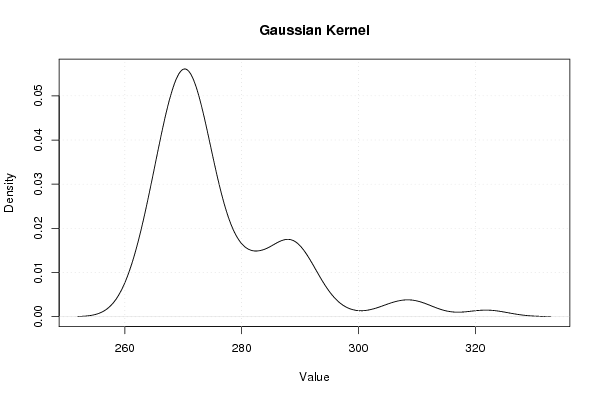PNG link Postscript link PDF link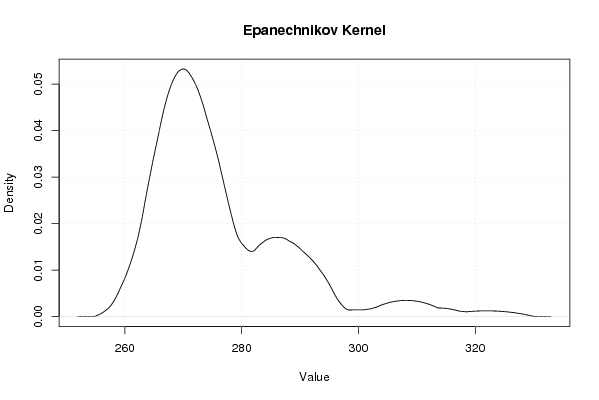PNG link Postscript link PDF link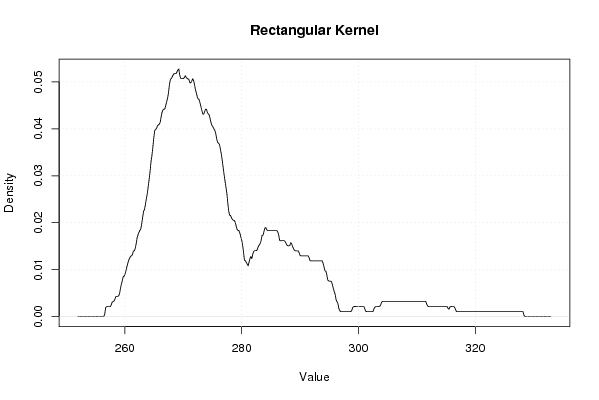PNG link Postscript link PDF link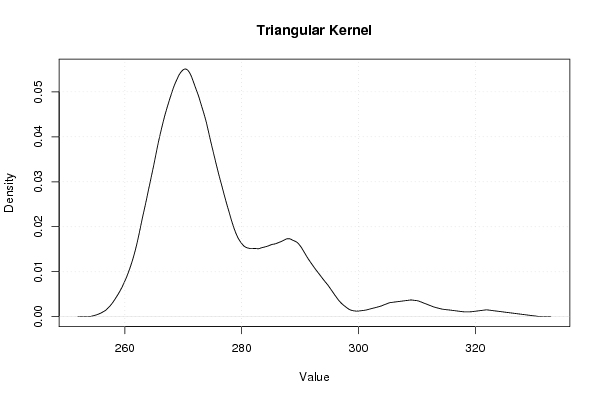PNG link Postscript link PDF link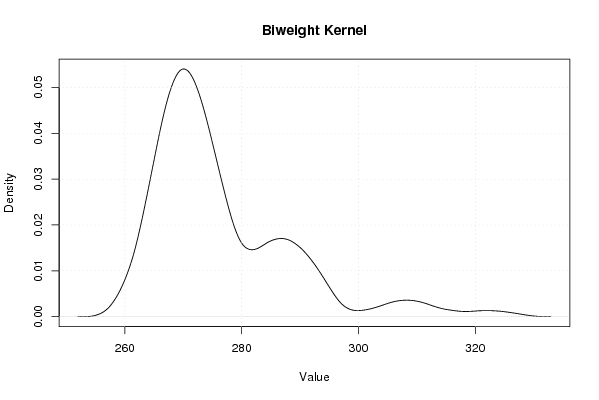PNG link Postscript link PDF link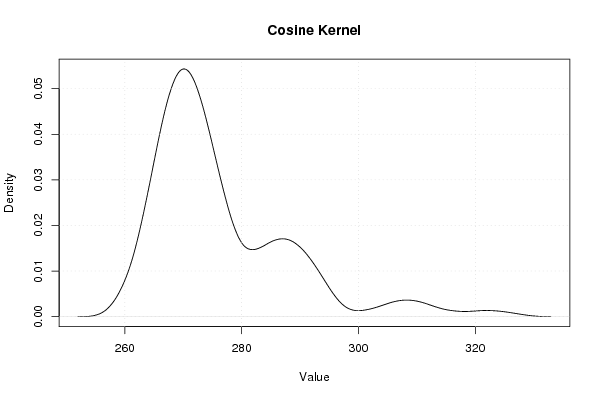PNG link Postscript link PDF link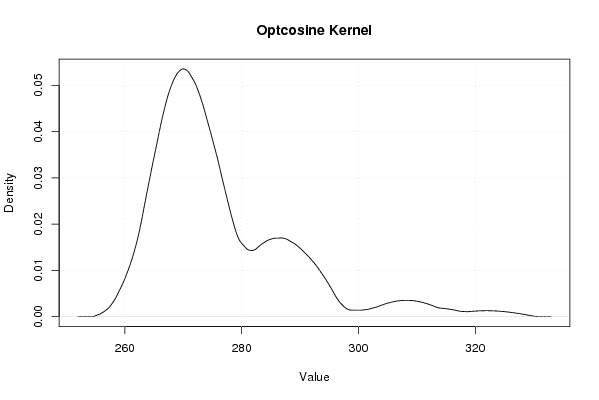PNG link Postscript link PDF link

Parameters (Session):
par1 = 0 ;
Parameters (R input):
par1 = 0 ;
R code (references can be found in the software module):
if (par1 == '0') bw <- 'nrd0'if (par1 != '0') bw <- as.numeric(par1)bitmap(file='density1.png')mydensity1<-density(x,bw=bw,kernel='gaussian',na.rm=TRUE)plot(mydensity1,main='Gaussian Kernel',xlab=xlab,ylab=ylab)grid()dev.off()mydensity1bitmap(file='density2.png')mydensity2<-density(x,bw=bw,kernel='epanechnikov',na.rm=TRUE)plot(mydensity2,main='Epanechnikov Kernel',xlab=xlab,ylab=ylab)grid()dev.off()bitmap(file='density3.png')mydensity3<-density(x,bw=bw,kernel='rectangular',na.rm=TRUE)plot(mydensity3,main='Rectangular Kernel',xlab=xlab,ylab=ylab)grid()dev.off()bitmap(file='density4.png')mydensity4<-density(x,bw=bw,kernel='triangular',na.rm=TRUE)plot(mydensity4,main='Triangular Kernel',xlab=xlab,ylab=ylab)grid()dev.off()bitmap(file='density5.png')mydensity5<-density(x,bw=bw,kernel='biweight',na.rm=TRUE)plot(mydensity5,main='Biweight Kernel',xlab=xlab,ylab=ylab)grid()dev.off()bitmap(file='density6.png')mydensity6<-density(x,bw=bw,kernel='cosine',na.rm=TRUE)plot(mydensity6,main='Cosine Kernel',xlab=xlab,ylab=ylab)grid()dev.off()bitmap(file='density7.png')mydensity7<-density(x,bw=bw,kernel='optcosine',na.rm=TRUE)plot(mydensity7,main='Optcosine Kernel',xlab=xlab,ylab=ylab)grid()dev.off()load(file='createtable')a<-table.start()a<-table.row.start(a)a<-table.element(a,'Properties of Density Trace',2,TRUE)a<-table.row.end(a)a<-table.row.start(a)a<-table.element(a,'Bandwidth',header=TRUE)a<-table.element(a,mydensity1$bw)a<-table.row.end(a)a<-table.row.start(a)a<-table.element(a,'#Observations',header=TRUE)a<-table.element(a,mydensity1$n)a<-table.row.end(a)a<-table.end(a)table.save(a,file='mytable.tab')